• 本题要求编写程序，计算序列 1 + 1/2 + 1/3 + … 的前N项之和。 输入格式: 输入在一行中给出一个正整数N。 输出格式: 在一行中按照“sum = S”的格式输出部分和的值S，精确小数点后6位。题目保证计算结果不...
求N分之一序列前N项和

本题要求编写程序，计算序列 1 + 1/2 + 1/3 + … 的前N项之和。

输入格式:
输入在一行中给出一个正整数N。

输出格式:
在一行中按照“sum = S”的格式输出部分和的值S，精确到小数点后6位。题目保证计算结果不超过双精度范围。

输入样例:
6
输出样例:
sum = 2.450000

#include <stdio.h>
int main()
{
int i,n;
double sum=0;
scanf("%d",&n);
for(i=1;i<=n;i++){
sum+=1.0/i;
}
printf("sum = %.6lf",sum);
return 0;
}

求奇数分之一序列前N项和

本题要求编写程序，计算序列 1 + 1/3 + 1/5 + … 的前N项之和。

输入格式:
输入在一行中给出一个正整数N。

输出格式:
在一行中按照“sum = S”的格式输出部分和的值S，精确到小数点后6位。题目保证计算结果不超过双精度范围。

输入样例:
23
输出样例:
sum = 2.549541

#include <stdio.h>
int main()
{
int n,i=1;
int k=1;
double sum=0;
scanf("%d",&n);
for(i=1;i<=n;i++){
sum+=1.0/k;
k+=2;
}
printf("sum = %.6lf",sum);
return 0;
}

求简单交错序列前N项和

本题要求编写程序,计算序列 1 - 1/4 + 1/7 - 1/10 + … 的前N项之和。

输入格式:
输入在一行中给出一个正整数N。

输出格式:
在一行中按照“sum = S”的格式输出部分和的值S，精确到小数点后三位。题目保证计算结果不超过双精度范围。

输入样例:
10
输出样例:
sum = 0.819

#include <stdio.h>
int main()
{
int n,i,k=1,q=4;
double sum;
scanf("%d",&n);
if(n%2==0){
for(i=1;i<=n/2;i++) {
sum+=1.0/k;
k+=6;
}
for(i=1;i<=n/2;i++){
sum-=1.0/q;
q+=6;
}
}
else{
for(i=1;i<=n/2+1;i++){
sum+=1.0/k;
k+=6;
}
for(i=1;i<=n/2;i++){
sum-=1.0/q;
q+=6;
}
}
printf("sum = %.3lf",sum);
return 0;
}

求平方与倒数序列的部分和

本题要求对两个正整数m和n（m≤n）编写程序，计算序列和
m​^2+1/m+(m+1)​ ^2​​ +1/(m+1)+⋯+n​ ^2​​ +1/n。

输入格式:
输入在一行中给出两个正整数m和n（m≤n），其间以空格分开。

输出格式:
在一行中按照“sum = S”的格式输出部分和的值S，精确到小数点后六位。题目保证计算结果不超过双精度范围。

输入样例:
5 10
输出样例:
sum = 355.845635

#include <stdio.h>
#include <math.h>
int main()
{
int m,n,i;
double sum;
scanf("%d %d",&m,&n);
for(i=m;i<=n;i++){
sum+=pow(i,2)+1.0/i;
}
printf("sum = %.6lf",sum);
return 0;
}

求交错序列前N项和

本题要求编写程序，计算交错序列 1-2/3+3/5-4/7+5/9-6/11+… 的前N项之和。

输入格式:
输入在一行中给出一个正整数N。

输出格式:
在一行中输出部分和的值，结果保留三位小数。

输入样例:
5
输出样例:
0.917

#include <stdio.h>
int main()
{
int n,i;
double k=1,p=3,u=1,y=2;
double sum=0,sum1=0,sum2=0;
scanf("%d",&n);
if(n%2==0){
for(i=1;i<=n/2;i++){
sum1+=(u/k)*1.0;
u+=2;
k+=4;
}
for(i=1;i<=n/2;i++){
sum2+=-(y/p)*1.0;
p+=4;
y+=2;
}
sum=sum1+sum2;
printf("%.3lf",sum);
}
else{
for(i=1;i<=n/2+1;i++){
sum1+=(double)(u/k);
u+=2;
k+=4;
}
for(i=1;i<=n/2;i++){
sum2+=-(double)(y/p);
p+=4;
y+=2;
}
sum=sum1+sum2;
printf("%.3lf",sum);
}
return 0;
}

求平方根序列前N项和

本题要求编写程序，计算平方根序列√
​1 +√​2​​ +√​3+⋯的前N项之和。可包含头文件math.h，并调用sqrt函数求平方根。

输入格式:
输入在一行中给出一个正整数N。

输出格式:
在一行中按照“sum = S”的格式输出部分和的值S，精确到小数点后两位。题目保证计算结果不超过双精度范围。

输入样例:
10
输出样例:
sum = 22.47

#include <stdio.h>
#include <math.h>
int main()
{
int n,i;
double sum;
scanf("%d",&n);
for(i=1;i<=n;i++){
sum=sum+sqrt(i);
}
printf("sum = %.2lf",sum);
return 0;
}

求阶乘序列前N项和

本题要求编写程序，计算序列 1!+2!+3!+⋯ 的前N项之和。

输入格式:
输入在一行中给出一个不超过12的正整数N。

输出格式:
在一行中输出整数结果。

输入样例:
5
输出样例:
153

#include <stdio.h>
int factorial(int n)
{
int i,result=1;
for(i=1;i<=n;i++){
result*=i;
}
return result;
}
int main()
{
int n,i,k;
scanf("%d",&n);
for(i=1;i<=n;i++){
k+=factorial(i);
}
printf("%d",k);
return 0;
}


乌拉乌拉无了无了
题目来自展开全文• target[i]=target[i-1]+source[i] //将左边正数累加当前目标数组； r++; //正数计数增加1 } } //循环完成，完成一组连续正数的累计求和，正数数量为z个 limit=limit-r; //经过正数求和，剩余待处理数据共...c
• 用while、for、do…while循环语句计算求和问题： #include<stdio.h>... i++)//循环i从1到1000 { item = sign * m*1.0 / n;//计算每一项 sign = -sign;//改变每一项的正负 m = m + n;//计算分
用while、for、do…while循环语句计算求和问题：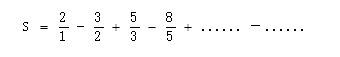#include<stdio.h>
int main()
{
double item, sum = 0;
int i, sign = 1;
double m = 2, n = 1;
for (i = 1; i <= 1000; i++)//循环i从1到1000
{
item = sign * m*1.0 / n;//计算每一项
sign = -sign;//改变每一项的正负
m = m + n;//计算分子
n = m - n;//计算分母
sum += item;//求和
}
printf("sum=%f\n", sum);//输出和
return 0;
}

运行结果：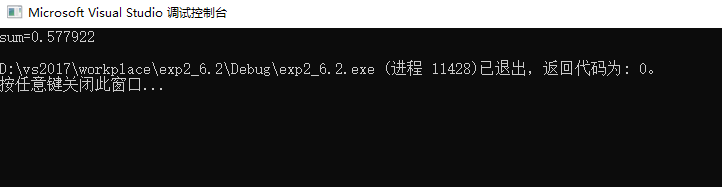展开全文• （23）教材第13章编程题第5题：编写程序实现对输入命令行参数求和。 说明：本题加深对命令行参数的理解、考查知识的综合应用。 （24）教材第16章编程题第5题：用结构变量解决搜索离用户输入时间最近的航班信息，并...
• 输入格式: 输入在一行中给出n(10≤n≤10000)和k(1≤k≤10)的值。 输出格式: 在一行中按下列格式输出: 素数1+素数2+…+素数k=总和值 其中素数按递减顺序输出。若n以内不够k个素数，则按实际个数输出。 输入...
• 序列求和：输入一个正实数eps，计算序列部分和 1 - 1/4 + 1/7 - 1/10 + ... ，精确最后一项的绝对值小于eps（保留6位小数）。 源程序（有错误的程序） #include<stdio.h> intmain() { int flag,n; double ...

一、改错题
题目
序列求和：输入一个正实数eps，计算序列部分和 1 - 1/4 + 1/7 - 1/10 + ... ，精确到最后一项的绝对值小于eps（保留6位小数）。
源程序（有错误的程序）
#include<stdio.h>
intmain()
{
int flag,n;
double eps,item,s;
printf("Input eps: ");
scanf("%f",&eps);
flag = 1;
s = 0;
n = 1;
do
{
item = 1/ n;
s = s + flag * item;
flag = -flag;
n = n + 3;
}
while( item < eps)
printf( "s = %f\n",s);
return0;
}
错误信息1：错误原因：while( item < eps)的后面没有加引号
改正方法：在后面加上引号
错误信息2：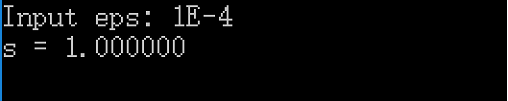错误原因：第八行输入格式有问题
改正方法：“%f”改成“%lf”
错误信息3：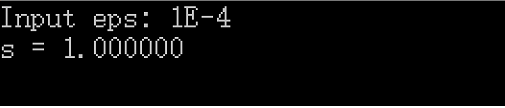错误原因：第13行中的运算为整型运算，不能出现实数
改正方法：将“item = 1/ n”中的“1”改成“1.0”，
错误信息4：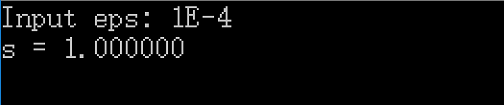错误原因：第17行中while()语句的括号里的表达式错误，与题目不符
改正方法：将“while( item > eps)”里的“<”改成“>”
错误信息5：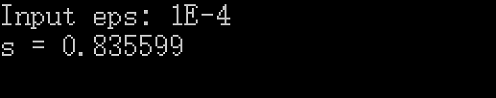错误原因：最后输出时格式符不正确，输出没有保留6位小数
改正方法：将“printf( "s = %f\n",s)”中的“%f”改成“%.6f”
改正后的源代码：
#include<stdio.h>
intmain()
{
int flag,n;
double eps,item,s;
printf("Input eps: ");
scanf("%lf",&eps);
flag = 1;
s = 0;
n = 1;
do
{
item = 1.0 / n;
s = s + flag * item;
flag = -flag;
n = n + 3;
}
while( item > eps);
printf( "s = %.6f\n",s);
return0;
}
二、学习总结
1.语句while(1)和for(;;)是什么意思？如何保证这种循环能正常执行？
1.while(1)表示无限循环
2.for(;;)也是无限循环
3.由于电脑判断时只判断1和0，所以只要非零就可以一直执行
2.针对上面三种情况，分别采用什么循环语句实现比较好？对每种情况，用我们完成的两次循环结构作业中的题目加以说明。
（1）循环次数已知，用for语句
（2）循环次数未知，但循环条件明确：使用while语句
（3）循环次数未知，且循环条件未知，需要在循环体中明确：使用do...while语句
3.有如下问题：输入一批学生成绩，以-1作为结束，计算学生的平均成绩。要求分别用for语句、while语句、do while语句三种循环语句实现，并说明你认为哪种形式更合适？
因为循环次数未知 所以用for就不太方便 循环条件非-1存在 所以循环条件明确 使用do while更合适
三、实验总结
1.求给定精度的简单交错序列部分和
（1）题目
本题要求编写程序，计算序列部分和 1 - 1/4 + 1/7 - 1/10 + ... 直到最后一项的绝对值不大于给定精度eps。
（2）流程图（3）源代码
#include<stdio.h>
#include<math.h>
intmain()
{
double eps,item,S = 0;
int denominator = 1,flag = 1;     item = 1.0;
scanf("%lf",&eps);
if(eps >= item)
{
S = 1.0;
}
while(fabs(item) > eps)
{
item = flag *1.0 / denominator;
S = S + item;
flag = -flag;
denominator = denominator + 3;
}

printf("sum = %.6f",S);
return0;
}
（4）实验分析
问题1：，输出结果错误，为0.750000
原因：item的绝对值不大于给定精度eps，while判断条件却是while(item > eps)
解决办法：把item改为它的绝对值
问题2：最后一项大于eps时输出结果错误
原因：丢了最后一项的绝对值大于等于给定精度eps时的情况
解决办法：在“scanf("%lf",&eps);”后面添加if语句
（5）本题PTA提交列表2.猜数字游戏
（1）题目
猜数字游戏是令游戏机随机产生一个100以内的正整数，用户输入一个数对其进行猜测，需要你编写程序自动对其与随机产生的被猜数进行比较，并提示大了（“Too big”），还是小了（“Too small”），相等表示猜到了。如果猜到，则结束程序。程序还要求统计猜的次数，如果1次猜出该数，提示“Bingo!”；如果3次以内猜到该数，则提示“Lucky You!”；如果超过3次但是在N（>3）次以内（包括第N次）猜到该数，则提示“Good Guess!”；如果超过N次都没有猜到，则提示“Game Over”，并结束程序。如果在到达N次之前，用户输入了一个负数，也输出“Game Over”，并结束程序。
（2）流程图（3）源代码
#include<stdio.h>
intmain()
{
int num,N,i,a;
i = 1;
scanf("%d %d",&num,&N);
do
{
scanf("%d",&a);
if(a <0)
{ printf("Game Over");
break;
}
elseif(a == num && i <= N)
{
if(i == 1)
{
printf("Bingo!");
break;
}
elseif(i <= 3)
{
printf("Lucky You!");
break;
}
elseif(i >3&& i <= N)
{
printf("Good Guess!");
break;
}
}
elseif(i > N)
{
printf("Game Over");
break;
}
elseif(a > num)
{
printf("Too big\n");
}
elseif(a < num)
{
printf("Too small\n");
}
i++;
}
while(num != a);
return0;
}
（4）实验分析
问题：当输入负数时没有停止
原因：if语句后没有break
解决办法：在后面加上break；
（5）本题PTA提交列表3.求奇数和
（1）题目
本题要求计算给定的一系列正整数中奇数的和。
（2）流程图（3）源代码
#include<stdio.h>
intmain()
{
int n,sum = 0;
do
{
scanf("%d",&n);
if (n % 2 == 1)
{
sum += n;
}
}
while (n >0);
printf("%d\n", sum);
return0;
}
（4）实验分析
问题1：编译错误
原因：if语句的括号内是判断语句而不是运算句
解决办法：加一个等号
（5）本题PTA提交列表博客互评
1.刘冀飞
代码格式输入有错误
2.吉炎臣
还是那个槽点 适合手机看
3.沐栀*
同学 你的作业 十分整齐 并且简洁 值得我学习

转载于:https://www.cnblogs.com/hfaq/p/7862345.html
展开全文• 意思是：i=1n+k就是要求和几次，即从1n+k）次，所得结果再乘以3，输出即可。 3． 慧慧拿奖学金 这道题其实看明白的很简单。 定义一个数组a，容量看题目要求；把慧慧的成绩以及...
2019级C语言程序设计新生第一次比赛题解
1．	You can really dance
很简单，暴力输出！这道题比的就是手速！
2．	想AK吗？
想做这道题就一定要明白 ‘求和’ 这个符号；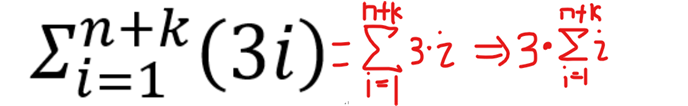意思是：i=1，n+k就是要求和几次，即从1加到（n+k）次，所得结果再乘以3，输出即可。
3．	慧慧拿奖学金
这道题其实看明白的很简单。
定义一个数组a，容量看题目要求；把慧慧的成绩以及班里其他n名同学的成绩输入到数组中，然后进行冒泡排序，按照从大到小的顺序排序，然后判断慧慧成绩（q）与第m个人的成绩相比（即a[m-1]）若不小a[m-1]即慧慧成绩在前m名输出“YES”；反之，输出“NO”， 之后取前m名的成绩输出即可，注意特判第m名成绩是否有重复，若有重复，（注意题目字眼！要求一并输出！即若第m名是88分m+1名也是88分，则都输出！），一并输出即可！
4．	今晚沙漠吃鸡
看好格式；假设在每站都加满油一旦两个加油站的路程超过油箱的容量，即不能达到下一个加油站，则意味“如果两个相邻的加油站之间的路程（a[ i+1 ]-a[ i ]）大于油箱容量（V）则输出‘-1’ ” 定义一个数组a 1000 ，先用for（）循环给数组赋值，给一个for()循环，让数组里面进行比较即算两个加油站的路程是否大于油箱容量，如图：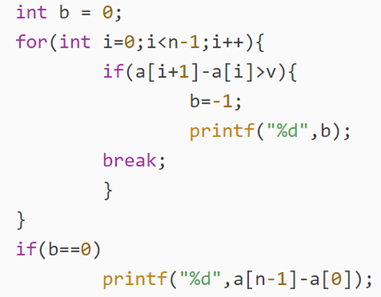这里的b代表是否能到达终点，若存在路程大于容量则为-1并输出；否则b还是0，即代表能够最终到达，那么最小的加油量即为第一个加油站到最后一个加油站的路程（ a[n-1]-a ）（汽油）。
5．	凯凯玩棋子
由题目可知，（x1，y1）在右下角（x2，y2）在右下角，把他想象成一个二维坐标轴，如图：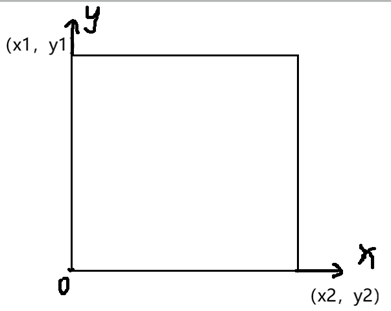简单明了，其他的就是注意多组输入输出和输出格式。
6．	A+B（V）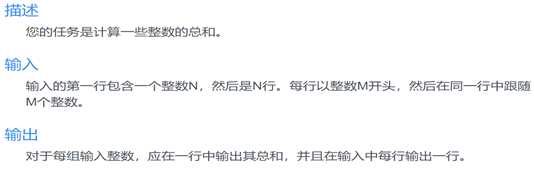这就是翻译过来的题目，很简单，直接求和吧。
ACM比赛可是不会让电脑翻译的，都是自己拿词典查的，所以说，少年，好好背单词吧！


展开全文• 拷贝tc编译器目录下，运行tc.exe，打开编译器， 按【F3】键或者“File->Open”菜单命令，打开1.c文件， 按【Ctrl+F9】键，或者“Run->Run”菜单命令，编译运行该程序。 ◎ 光盘目录清单如下： 第一...
• 序列求和：输入一个正实数eps，计算序列部分和 1 - 1/4 + 1/7 - 1/10 + ... ，精确最后一项的绝对值小于eps（保留6位小数）。 　输入输出样例： 　Input eps：1E-4 　s = 0.835699 　 源程序（有错误的程序） #...
• 序列求和：输入一个正实数eps，计算序列部分和 1 - 1/4 + 1/7 - 1/10 + ... ，精确最后一项的绝对值小于eps（保留6位小数）。 　输入输出样例： 　Input eps：1E-4 　s = 0.835699 　 源程序（有错误的程序） #...
• 现给定一个实数x，要求利用此幂级数部分和求e​x​​的近似值，求和一直继续最后一项的绝对值小于0.00001。 输入格式: 输入在一行中给出一个实数x∈[0,5]。 输出格式: 在一行中输出满足条件的幂级数部分和，保留...算法
• 序列求和：输入一个正实数eps，计算序列部分和 1 - 1/4 + 1/7 - 1/10 + ... ，精确最后一项的绝对值小于eps（保留6位小数）。 　输入输出样例： 　Input eps：1E-4 　s = 0.835699 　 源程序（有错误的程序） #...
• 序列求和：输入一个正实数eps，计算序列部分和 1 - 1/4 + 1/7 - 1/10 + ... ，精确最后一项的绝对值小于eps（保留6位小数）。 修改前的源代码 错误信息（1）： 错误原因：用do while循环语句在while后面要加分号...
• 题目分析： 该题是对输入的一个大数的各位数之和求和，简单来看可以将该数取余后进行分离各位数的操作，就可以到达题目要求。...C语言程序： #include<stdio.h> int main() { int n,num; ...CSP
• ## C语言求完数

千次阅读 2018-09-13 20:20:04
3.将当前数字的因子分解出来,累加当前因子求和,将这个数的所有因子保存数组a中  4.用因子的和与当前数字对比,若相等即是完数,输出这个完数和它的因子 5.刷新当前数据,使sum和数组a恢复初始状态以便于下一个数字...# c语言求和1到n程序c语言 订阅